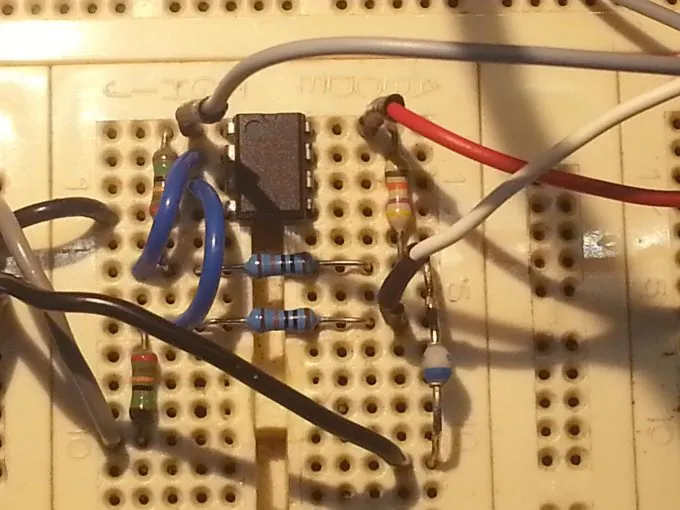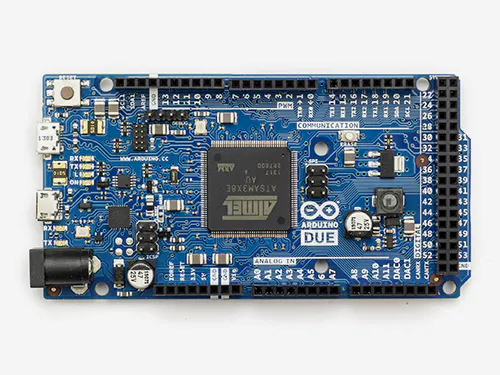Project tutorialHow to modify analog output range of Arduino Due © GPL3+

With this simple circuit the analog output of Arduino Due start from zero

• 18,098 views
• 17 respects

Components and suppliesArduino Due
×1
 C1, C2 capacitor, 100 nF, ceramic AVX or polyester
×1
 R1 resistor, 100 kΩ, ±1%, ¼ W
×2
 R2 resistor, 150 kΩ, ±1%, ¼ W
×2
 R3 resistor, 1.3 kΩ, ±1%, ¼ W
×1
 R4 resistor, 6.8 kΩ, ±1%, ¼ W
×1
 Rp resistor trimmer, 200 Ω, multi turn is better
×1
 U1 LM358 (see text)
×1

This project aims to change the analog output of Arduino Two in order to have a rangeequal to the input.

Arduino Due does not have an analog output voltage from 0 V to Vref, but from 1/6 to 5/6 of the reference

voltage, that is, 0.55 V and 2.75V with Vref = 3.3 V. This is also confirmed by the Atmel (see bibliography 1).

The output voltage range of the DAC is only 2.75-0.55 = 2.2 V, with a resolution of 2.2 /4095 = 0.5372 mV.

A simple differential amplifier, realized with an op amp, is used to remove the 0.55 V offset and to amplify

the output signal in order to reach the desired value. The figure 1 shows the scheme of this circuit.

To generate the voltage Vp = 0.55 V, that is, the minimum value of the DAC, is used the divider formed by

R3, R4 and Rp. The trimmer pot Rp is used to adjust Vp in order to have an output value close to 0 V in

correspondence with the number 0 sent to the DAC. With a single power supply you can never get exactly

0V but you approach some mV, about 8 in my prototype.

The differential amplifier has the following gain:

Just change the gain, for a different output voltage, but less than 4 V,.

With a gain G = 3.3 /2.2 = 1.5, the output of this circuit has the desired range from 0 to 3.3V.

G = Vo/(Vi-Vp) = R2 / R1

It is recommended to use resistors with a tolerance of 1% or less, particularly for the four resistors of the

amplifier. Don’t use operational amplifier as LM741, LM1458, TL081 and other that are not suitable for

single-supply. If it is required an output maximum voltage above 3.3 V it is essential to use a rail to rail op

amp as TLC2272 or equivalent.

SchematicsAnalog XY-Plotter Output to HP-GL Translator

Project showcase by johan34

• 4,193 views
• 17 respects

Project tutorial by Giovanni Carrera

• 4,986 views
• 3 respects

Analog Clock using 1Sheeld Graphical LCD

Project tutorial by Ahmed El-Hinidy

• 2,069 views
• 7 respects

Ultrasonic Range Detector With Arduino

Project tutorial by Salman Faris

• 27,203 views
• 25 respects

Ultrasonic Range Finder

Project tutorial by Saaket Poray

• 9,418 views